# KR20130055341A - Apparatus for converting signal of power metering system, power metering system and method for converting signal of power metering system - Google Patents

Apparatus for converting signal of power metering system, power metering system and method for converting signal of power metering system Download PDF

## Info

Publication number
KR20130055341A
KR20130055341A KR1020110121039A KR20110121039A KR20130055341A KR 20130055341 A KR20130055341 A KR 20130055341A KR 1020110121039 A KR1020110121039 A KR 1020110121039A KR 20110121039 A KR20110121039 A KR 20110121039A KR 20130055341 A KR20130055341 A KR 20130055341A
Authority
KR
South Korea
Prior art keywords
signal
frequency
digital
current
down
Prior art date
Application number
KR1020110121039A
Other languages
Korean (ko)
Inventor
강신재
Original Assignee
삼성전기주식회사
한국전력공사
Priority date (The priority date is an assumption and is not a legal conclusion. Google has not performed a legal analysis and makes no representation as to the accuracy of the date listed.)
Filing date
Publication date
Application filed by 삼성전기주식회사, 한국전력공사 filed Critical 삼성전기주식회사
Priority to KR1020110121039A priority Critical patent/KR20130055341A/en
Publication of KR20130055341A publication Critical patent/KR20130055341A/en

• 238000006243 chemical reaction Methods 0 abstract 3

## Images

•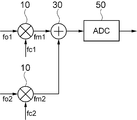•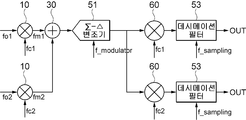•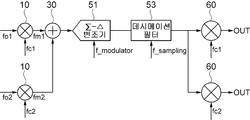•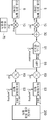•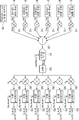••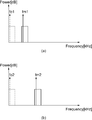•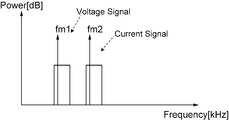•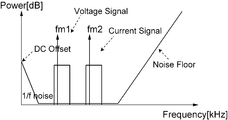•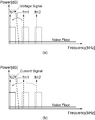•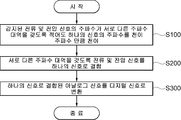•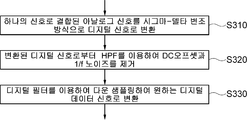•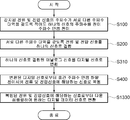•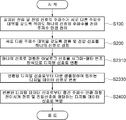## Classifications

• HELECTRICITY
• H03BASIC ELECTRONIC CIRCUITRY
• H03MCODING; DECODING; CODE CONVERSION IN GENERAL
• H03M1/00Analogue/digital conversion; Digital/analogue conversion
• H03M1/002Provisions or arrangements for saving power, e.g. by allowing a sleep mode, using lower supply voltage for downstream stages, using multiple clock domains or by selectively turning on stages when needed
• GPHYSICS
• G01MEASURING; TESTING
• G01RMEASURING ELECTRIC VARIABLES; MEASURING MAGNETIC VARIABLES
• G01R21/00Arrangements for measuring electric power or power factor
• G01R21/06Arrangements for measuring electric power or power factor by measuring current and voltage
• HELECTRICITY
• H03BASIC ELECTRONIC CIRCUITRY
• H03MCODING; DECODING; CODE CONVERSION IN GENERAL
• H03M3/00Conversion of analogue values to or from differential modulation
• H03M3/30Delta-sigma modulation
• H03M3/458Analogue/digital converters using delta-sigma modulation as an intermediate step
• HELECTRICITY
• H03BASIC ELECTRONIC CIRCUITRY
• H03MCODING; DECODING; CODE CONVERSION IN GENERAL
• H03M3/00Conversion of analogue values to or from differential modulation
• H03M3/30Delta-sigma modulation
• H03M3/458Analogue/digital converters using delta-sigma modulation as an intermediate step
• H03M3/466Multiplexed conversion systems
• H03M3/472Shared, i.e. using a single converter for multiple channels
• GPHYSICS
• G01MEASURING; TESTING
• G01RMEASURING ELECTRIC VARIABLES; MEASURING MAGNETIC VARIABLES
• G01R21/00Arrangements for measuring electric power or power factor
• G01R21/133Arrangements for measuring electric power or power factor by using digital technique

## Abstract

The present invention relates to a signal conversion device, a power meter system, and a signal conversion method of a power meter system. According to one embodiment of the invention, the frequency shifting unit for shifting the frequency of the at least one signal by a transition frequency so that the sensed current and voltage signals have different frequency bands; A signal combiner for combining current and voltage signals having different frequency bands into one signal; And an analog-digital converter for converting the analog signal combined into one signal into a digital signal. An apparatus for converting a signal of a watt-hour system comprising a is proposed. In addition, a power meter system including the same and a signal conversion method of the power meter system are proposed.

## Description

Signal conversion device of power meter system, signal conversion method of power meter system and power meter system {APPARATUS FOR CONVERTING SIGNAL OF POWER METERING SYSTEM, POWER METERING SYSTEM AND METHOD FOR CONVERTING SIGNAL OF POWER METERING SYSTEM}

The present invention relates to a signal conversion device, a power meter system, and a signal conversion method of a power meter system. More specifically, the present invention relates to a signal conversion device, a power meter system, and a signal conversion method of a power meter system of up-converting a frequency of a current and voltage signal, combining and processing them into one signal.

Recently, research on smart grid technology through convergence of information communication technology and power technology has been actively conducted. In order to implement a smart grid, an electronic electricity meter technology that accurately measures power consumption and converts it into digital information and a communication technology that transmits the converted digital information are required.

Electronic electricity meters generally consist of signal sensing blocks, signal conversion blocks, arithmetic blocks, and control blocks. Conventionally, two (voltage and current) or six ( Analog signals are converted into digital signals through signal conversion blocks (ADC) of three phase voltage and current). This can result in a large system area and high current consumption.

In the related art, an analog-to-digital converter is provided for each of the single-phase or three-phase voltage and current signals, so that a large area system is required, and current consumption is high.

The present invention is to solve this conventional problem, by up-converting the frequency of the current and voltage signal to combine and process as a single signal, it is possible to perform a signal conversion process with one analog-to-digital converter.

In addition, since the input frequency band of the analog-to-digital converter is a low frequency band (DC to 2KHz), there have been problems such as degradation of the resolution of the ADC due to DC offset and 1 / f noise and reduction of SNR. According to an embodiment, the analog-to-digital conversion process shifts the frequency to a frequency band that is not affected by DC offset and 1 / f noise or decreases to process the analog-to-digital conversion.

In order to solve the above problem, according to the first embodiment of the present invention, the frequency shifting unit for shifting the frequency of the at least one signal by the transition frequency so that the sensed current and voltage signals have different frequency bands; A signal combiner for combining current and voltage signals having different frequency bands into one signal; And an analog-digital converter for converting the analog signal combined into one signal into a digital signal. An apparatus for converting a signal of a watt-hour system comprising a is proposed.

In another example of the present invention, the frequency shifting unit may shift the sensed current and voltage signals by different transition frequencies, respectively.

In another example, the current and voltage signals can be three phase signals.

In addition, according to one example, the frequency shifter is a frequency synthesizer for generating a frequency shifted intermediate frequency by synthesizing the frequency of both signals and different transition frequencies so that the current and voltage signals have different frequency bands, and the signal The combiner may be a power combiner.

Further, in one example, the analog-to-digital converter comprises: a sigma-delta modulator for converting an analog signal combined into one signal into a digital signal; And a digital filter which down-samples the converted digital signal into a desired digital data signal. . &Lt; / RTI &gt;

At this point, in one example, the digital filter is a decimation filter, and the decimation filter may convert the converted digital signal into a desired N-bit digital data signal by low pass band filtering and down sampling.

Also, in one example, the analog-digital converter may further include a bandpass filter that removes and outputs a low frequency band DC offset and 1 / f noise component from the digital signal converted by the sigma-delta modulator.

In another example, the digital filter further includes a frequency down converter for shifting down from the digital signal converted in the sigma-delta modulator to a signal corresponding to the current and voltage signals, and the digital filter is restored in the frequency down converter. Down sampling from current and voltage signals can be converted to desired digital data signals.

In another example, the frequency converter may further include a frequency down converter for shifting down from the converted digital data signal by a shift frequency to restore current and voltage signals.

Next, to solve the above problem, according to a second embodiment of the present invention, the current and voltage sensing block for sensing the current and voltage signals; A signal conversion device of the power meter system according to any one of the first embodiments of the present invention, which converts a signal sensed by the sensing block into a digital signal; And a digital processing block for calculating an amount of power from the digital data value output from the signal conversion device. Comprising, a wattmeter system is proposed.

Further, in one example, the analog-to-digital converter of the signal conversion device includes: a sigma-delta modulator for converting an analog signal combined into one signal into a digital signal; And a digital filter which down-samples the converted digital signal into a desired digital data signal. The apparatus for converting a signal further includes a frequency down converter for shifting down from a digital signal converted by a sigma-delta modulator by a transition frequency to restore a signal corresponding to a current and voltage signal, and the digital filter includes a frequency down converter. Down sampling from the recovered current and voltage signals can be converted to a desired digital data signal.

Also, in another example, the analog-to-digital converter of the signal conversion device includes: a sigma-delta modulator for converting an analog signal combined into one signal into a digital signal; And a digital filter which down-samples the converted digital signal into a desired digital data signal. The signal converting apparatus may further include a frequency down converter configured to recover the current and voltage signals by shifting down from the converted digital data signal by a transition frequency.

Next, in order to solve the above problem, according to the third embodiment of the present invention, the step of shifting the frequency of the at least one signal by the transition frequency so that the sensed current and voltage signals have different frequency bands; Combining the current and voltage signals having different frequency bands into one signal; And converting the analog signal combined into one signal into a digital signal. A signal conversion method of a watt-hour system comprising a is proposed.

In another example of the present invention, in the step of shifting the frequency, the sensed current and voltage signals may be shifted by different transition frequencies.

In another example, the current and voltage signals can be three phase signals.

Further, in one example, the step of converting to a digital signal includes: a sigma-delta modulation step of converting an analog signal combined into one signal into a digital signal in a sigma-delta modulation scheme; And down-sampling the digital signal converted in the sigma-delta modulation step using a digital filter to a desired digital data signal. . &Lt; / RTI &gt;

According to another example, the digital filter is a decimation filter, and in the step of converting into a digital data signal, the digital filter is converted into a desired N-bit digital data signal by low pass band filtering and down sampling using the decimation filter. can do.

According to one example, the converting into a digital signal may include outputting a low frequency band DC offset and a 1 / f noise component by using a band pass filter from the digital signal converted in the sigma-delta modulation step. It may further include.

Furthermore, according to one example, the method further includes a frequency down converting step of shifting down from the digital signal converted in the sigma-delta modulation step by a transition frequency to restore the signal corresponding to the current and voltage signals, and the digital filter further includes a frequency down conversion. In the converting step, it is down-sampled from the restored current and voltage signals and converted into a desired digital data signal.

In another example, the method may further include a frequency down-converting step of recovering the current and voltage signals by shifting down from the converted digital data signal by a transition frequency.

According to an embodiment of the present invention, by converting the frequency of the current and voltage signal to combine and process in one signal, two or six voltage and current signals in a single-phase or three-phase power system as one analog-digital Conversion can be done through the converter, thereby reducing power consumption and system size.

In addition, according to one embodiment of the present invention, by processing the analog-to-digital conversion by shifting the frequency of the sensed current and voltage signal to different frequency bands, it is possible to minimize the interference between different frequencies or different phases .

In addition, according to one embodiment of the present invention, by processing the analog-to-digital conversion by shifting the frequency of the sensed current and voltage signal to different frequency bands, the DC offset and 1 / f noise Unaffected or reduced to a minimum, this allows sampling of signals with high SNR.

It is apparent that various effects which are not directly mentioned according to various embodiments of the present disclosure may be derived by those skilled in the art from various configurations according to the embodiments of the present disclosure.

1 is a block diagram schematically illustrating an apparatus for converting a signal of a power meter system according to an embodiment of the present invention.
2A and 2B are block diagrams schematically illustrating a signal conversion apparatus of a power meter system according to another embodiment of the present invention.
3 is a block diagram schematically illustrating a power meter system including a signal conversion device of a power meter system according to another embodiment of the present invention.
4 is a block diagram schematically illustrating an apparatus for converting a signal of a power meter system according to another embodiment of the present invention.
5 is a diagram illustrating a signal component and a transition frequency detected by a sensing block according to an embodiment of the present invention.
FIG. 6 is a diagram illustrating a signal component transitioned by a frequency shifting unit in an embodiment of the present invention.
7 is a diagram illustrating signal components coupled by a signal combiner in an embodiment of the present invention.
8 is a diagram showing an output frequency spectrum by a sigma-delta modulator according to an embodiment of the present invention.
9 is a diagram illustrating signal components processed by a frequency down converter and a digital filter in an embodiment of the present invention.
10 is a flowchart schematically illustrating a signal conversion method of a power meter system according to another embodiment of the present invention.
11 is a flowchart schematically illustrating some processes of a signal conversion method of a power meter system according to an embodiment of the present invention.
12 is a flowchart schematically illustrating a signal conversion method of a power meter system according to another embodiment of the present invention.
FIG. 13 is a flowchart schematically illustrating a signal conversion method of a power meter system according to another embodiment of the present invention.

Embodiments of the present invention for achieving the above object will be described with reference to the accompanying drawings. In the description, the same reference numerals denote the same components, and a detailed description may be omitted for the sake of understanding of the present invention to those skilled in the art.

As used herein, unless an element is referred to as being 'direct' in connection, combination, or placement with other elements, it is to be understood that not only are there forms of being 'directly connected, They may also be present in the form of being connected, bonded or disposed. In addition, the same is true when terms including 'contact' such as 'up', 'up', 'lower', 'below' are included. Directional terms may be construed to encompass corresponding relative directional concepts as the reference element is inverted or its direction is changed.

It should be noted that, even though a singular expression is described in this specification, it can be used as a concept representing the entire plurality of constitutions unless it is contrary to, or obviously different from, or inconsistent with the inventive concept. It is to be understood that the description of 'comprising', 'having', 'comprising', 'comprising', etc., in this specification includes the possibility of the presence or addition of one or more other components or combinations thereof.

First, a signal conversion apparatus of a power meter system according to a first embodiment of the present invention will be described in detail with reference to the accompanying drawings.

1 is a block diagram schematically showing a signal conversion device of a power meter system according to an embodiment of the present invention, Figures 2a and 2b is a schematic diagram of a signal conversion device of a power meter system according to another embodiment of the present invention 3 is a block diagram schematically illustrating a wattmeter system including a signal conversion device of a wattmeter system according to another embodiment of the present invention, and FIG. 4 is a block diagram of another embodiment of the present invention. A block diagram schematically showing a signal conversion device of a watt-hour meter system. FIG. 5 is a diagram illustrating a signal component and a transition frequency detected by a sensing block according to an embodiment of the present invention. FIG. 6 is a diagram illustrating a signal component transitioned by a frequency transition unit according to an embodiment of the present invention. In the embodiment of the present invention is a view showing a signal component coupled by a signal combiner, Figure 8 is a view showing the output frequency spectrum by the sigma-delta modulator in an embodiment of the present invention, Figure 9 is an embodiment of the present invention In the example, the signal components processed by the frequency down converter and the digital filter are shown.

Referring to FIG. 1, a signal conversion device of a watt-hour system according to a first embodiment of the present invention includes a frequency shifter 10, a signal combiner 30, and an analog-digital converter 50.

The frequency shifter 10 shifts the frequency of at least one signal by a transition frequency so that the sensed current and voltage signals have different frequency bands. By shifting the frequency of at least one signal by a transition frequency so that the current and voltage signals have different frequency bands in the frequency shifter 10 to have an intermediate frequency, the frequency shifter 10 can be combined into one signal without collision of the frequency band. . For example, in the case of a single-phase system, a frequency band in which the current and voltage signals are different from each other by shifting the frequency of one of the current and voltage signals by a transition frequency or by a different transition frequency with respect to the frequency of the current and voltage signals. It can be to have. At this time, the fundamental frequency signal (~ 60Hz) of the detected voltage and current signal is a voltage reference block for supplying a reference voltage required for signal conversion and DC offset and 1 / f noise of a signal conversion block such as an ADC. It is necessary to shift the frequency as it will degrade due to the effect, causing degradation of accuracy, which is an important characteristic of the power metering system. In this case, the transition frequency may be a frequency capable of shifting to a frequency band not affected by DC offset and 1 / f noise in the low frequency band during the analog-digital conversion process.

In addition, referring to FIG. 1, in one example, the frequency shifter 10 may shift the sensed current and voltage signals by different transition frequencies. According to the present embodiment, resolution of analog-to-digital conversion due to DC offset and 1 / f noise in the low frequency band during the analog-to-digital conversion process is performed by shifting each of the sensed current and voltage signals by different transition frequencies. ) Deterioration and SNR reduction can be prevented.

In general, the power line signal has characteristics of 110V or 220V strength and a fundamental frequency of 60Hz. At this time, harmonic components are generated due to the nonlinear characteristics of the power supply source and the power supply line path. In general, since the wattmeter analyzes up to the 20th harmonic component (1.2KHz), an intermediate frequency of the frequency shifted voltage signal and the current signal may have a transition interval of, for example, 1.2KHz or more.

3 and 5, current and voltage sensing blocks 5 and 6 detect current and voltage signals on power lines. At this time, the current signal and the voltage signal can be detected in the form of a voltage signal, and the magnitude of the corresponding voltage signal is attenuated and adjusted in the sensing block at a constant ratio to match the input range for the analog-digital conversion, respectively. For example, the current signal sensing block 6 may be detected as a voltage signal through a current transformer CT, a shunt resistor, a hall sensor, or the like. In order to prevent the frequency from overlapping when the current signal and the voltage signal detected by the current and voltage sensing blocks 5 and 6 are combined, the frequency shifter 10 transitions the current signal and the voltage signal by different transition frequencies, Transition one of the voltage signals by a transition frequency.

1 to 3, in one example, the frequency shifter 10 synthesizes the frequency and the transition frequency of at least one signal or the frequency of both signals such that the current and voltage signals have different frequency bands. And a frequency synthesizer 10 for synthesizing different transition frequencies with each other to generate a frequency shifted intermediate frequency.

5 and 6, the frequency synthesizer 10 has a voltage having a fundamental frequency component (DC ˜2 KHz) of fo1 and fo2 sensed by the sensing blocks 5 and 6 of respective voltages and currents. Each signal of the overcurrent is combined with a signal having a transition frequency of fc1 and fc2 generated by the frequency generator 70 to generate a signal corresponding to the intermediate frequency of fm1 and fm2. do. Since the fundamental frequency components of fo1 and fo2 detected by the sensing blocks 5 and 6 exist in the low frequency band, the transition frequency is prevented from being affected by the DC offset and 1 / f noise in the low frequency band during the analog-to-digital conversion process. You can make as many transitions. Where fm1 is fo1 + fc1 and fm2 is fo2 + fc2. That is, for example, the voltage and current detection signal having a fundamental frequency f0 of 60 Hz is up-converted by the frequency synthesizer 10 to the transition frequencies of fc1 and fc2, and thus the frequency of fm1 and fm2 as shown in FIG. It generates a voltage and current conversion signal with an intermediate frequency. In Figure 5, fo1 is the fundamental frequency of the sensed voltage signal, fo2 is the fundamental frequency of the sensed current signal. At this time, since the fundamental frequency is the same frequency of the 50 or 60 Hz band, it is necessary to shift with different transition frequencies. In order to make the frequency band different, a transition frequency fc1 for a voltage signal, for example, a 1 KHz frequency and a transition frequency fc2 for a current signal, for example, a 2 KHz frequency, is respectively synthesized with a fundamental frequency, as shown in FIG. The frequency is converted into an intermediate frequency fm1, for example, 1.06 KHz, and an intermediate frequency fm2, eg, 2.06 KHz, for the current signal. In FIG. 6, the intermediate frequency fm1 for the voltage signal and the intermediate frequency fm2 for the current signal may be combined into one signal because they have different frequency bands. In this case, the fundamental frequencies fo1 and fo2 have been described, but the sensed voltage signal and the current signal may include harmonic components in addition to the fundamental frequency, and these harmonic components may be frequency shifted integrally and combined into one signal together. Can be. In addition, 1KHz of the transition frequency fc1 and 2KHz of the transition frequency fc2 are exemplified to aid the understanding of the invention and do not limit the scope of the invention.

Since the analog-to-digital conversion is performed in each frequency band through frequency conversion according to the present embodiment, interference between different frequency bands or different phases can be minimized.

In one example, the current and voltage signals may be single phase, or may be three phase signals as shown in FIG. In the three-phase system, the frequency shifter 10 shifts the frequency so that each of the three-phase voltage and current signals has a different frequency band. In this case, as shown in FIG. 4, all three phase voltage and current signals are shifted by different transition frequencies, or although not shown, except for any one of the three phase voltage and current signals, the remaining signals are different from each other. By shifting by enough, all three phase voltage and current signals can have different frequency bands.

In the case of a three-phase signal, since the frequency synthesizer 10 is required for each phase, for example, in the case of six or three-phase four-wire, seven frequency synthesizers 10 may be required. Alternatively, only five frequency synthesizers 10 may be provided when the remaining signals are shifted by different transition frequencies except for one of the three phase voltage and current signals. Referring to Fig. 4, a case of a three-phase signal is shown. For example, six frequency synthesizers 10 are shown.

In FIG. 1, the signal combiner 30 combines current and voltage signals having different frequency bands into one signal.

1 to 3, in one example, the signal combiner 30 may be a power combiner 30. In FIG. 1, for example, the frequency shifted voltage signal intermediate frequency fm1 and the frequency shifted current signal intermediate frequency fm2 are combined into one signal by the power combiner 30. Referring to FIG. 4, in the case of a three-phase signal, for example, six frequency signals transitioned from six frequency synthesizers 10 to different frequencies are combined into one signal having different frequency bands in one power combiner 30. Can be. Referring to FIG. 7, the power combiner 30 generates one signal having a frequency spectrum of the frequency shifted voltage signal intermediate frequency fm1 and the frequency shifted current signal intermediate frequency fm2.

Next, in FIG. 1, the analog-to-digital converter 50 converts an analog signal combined into one signal into a digital signal. The analog-digital converter 50 may include an analog-digital converter (ADC) and a filter. The analog-to-digital converter converts one combined analog signal into a digital signal, and the converted digital signal is passed through a filter to remove noise and obtain only a required frequency band signal. In general, analog-to-digital converters for power meters have a resolution of more than 15 bits.

In general, power line signals have characteristics of 110V or 220V strength and frequency of 60Hz. At this time, harmonic components are generated due to the nonlinear characteristics of the power supply source and the power supply line path. In general, since the wattmeter analyzes up to the 20th harmonic component (1.2KHz), the analog-to-digital converter 50 includes the fundamental frequency of the frequency shifted current and voltage included in one signal coupled to the signal combiner 30. In order to convert an analog signal consisting of a signal and harmonic components into a digital signal, an N bit digital signal having a specific sampling frequency (fSampling, Sampling Frequency) is output. In this case, in order to obtain characteristics such as high accuracy and high sensitivity, a sigma-delta (Sigma-Delta, Σ-Δ) method may be used. The sigma-delta scheme consists of a sigma-delta modulator 51 for modulation and a decimation filter 53 for demodulation.

According to the present embodiment, for example, two or six voltage and current signals may be converted through one analog-to-digital converter 50 in single-phase and three-phase power systems, thereby reducing power consumption and system size. Can be.

In addition, in one example, the analog-to-digital converter 50 may include a sigma-delta modulator 51 and a digital filter 53. At this time, the sigma-delta modulator 51 modulates the analog signal combined into one signal in a sigma-delta method and converts it into a digital signal. The digital filter 53 down-samples the converted digital signal into a desired digital data signal. Referring to FIG. 8, the output frequency spectrum of the sigma-delta modulator 51 for one signal having a frequency shifted voltage signal intermediate frequency fm1 and a frequency shifted current signal intermediate frequency fm2 band is shown. At this time, DC offset and 1 / f noise are formed in the low frequency band, and a high noise frequency spectrum is generated in the high frequency band compared to the low frequency band by a unique noise transfer function.

2A, 2B, 3, and 4, in one example, the digital filter 53 may be a decimation filter. The decimation filter 53 may convert the converted digital signal into a desired N-bit digital data signal by low pass band filtering and down sampling.

Here, the decimation filter 53 may include filters such as CIC, Sinc and Half-Band. The decimation filter 53 can remove the noise of the high frequency band by the noise transfer function (NTF) of the sigma-delta modulator 51 shown in FIG. In addition, the decimation filter 53 generates output data having a sampling rate suitable for OSR (Over Sampling Ratio).

Specifically, an analog signal composed of fundamental frequency signals and harmonic components of frequency shifted current and voltage included in one signal coupled to the signal combiner 30 is input to the sigma-delta modulator 51. In this case, the sigma-delta modulator 51 may sample and modulate the digital signal of 1 bit by sampling and modulating it at a period of over sampling frequency (fM). The modulated signal of 1 bit oversampled than the actual signal frequency is demodulated by the decimation filter 53 and restored to a desired sampling frequency (fSampling). In this case, the oversampling frequency is a few MHz band and the sampling frequency may have a band of about 2KHz ~ 10KHz. In general, the sampling frequency may have a frequency greater than or equal to 2.4 KHz, which is twice the signal of the frequency band fBW including a fundamental frequency component of 60 Hz and first to 20th harmonic components (120 Hz to 1.2 KHz). Since the current and voltage signals which are frequency shifted in the signal combiner are included in one signal, the sampling frequency may be determined in consideration of the transition frequency. for example,

In addition, referring to FIG. 3, in one example, the analog-to-digital converter 50 removes the low frequency band DC offset and 1 / f noise component from the digital signal converted by the sigma-delta modulator 51 and outputs the noise. A band pass filter 55 may be further included. In this case, the band pass filter 55 may be a high pass filter (HPF) 55 or a band pass filter (BPF). In FIG. 8, DC offset and 1 / f noise formed in the low frequency band may be removed while passing through the high pass filter (HPF) 55 or the band pass filter (BPF). Although not shown in FIGS. 2A and 2B, a bandpass filter 55 may be provided after the sigma-delta modulator 51.

According to the present embodiment, sampling of a signal having a higher SNR is possible without being affected by the DC offset and 1 / f noise by the sigma-delta modulator 51.

Also, referring to FIGS. 2A and 2B, a signal conversion device of a watt-hour system may further include a frequency down converter 60.

Referring to FIG. 2A, the frequency down converter 60 shifts down from the digital signal converted by the sigma-delta modulator 51 by a transition frequency to restore a signal corresponding to the current and voltage signals. In this case, the frequency down converter 60 may restore the current or voltage signal by shifting down the frequency-shifted current or voltage signal by a transition frequency. At this time, although not shown, in one example, a multiplexer may be inserted in front of the frequency down converter 60 for time division processing for each frequency.

In this case, referring to FIG. 2A, the digital filter 53 may down-sample the current and voltage signals restored by the frequency down converter 60 to convert the desired digital data signal. If there is a signal that does not have a frequency shifted, the frequency down converter 60 does not go through the process of shifting down as much as the transition frequency and separates and inputs the digital filter 53 to directly down-sample the digital filter 53. Can be converted into the desired digital data signal.

The frequency down converter 60, for example, shifts the voltage signal and the current signal by the high pass filter downward by the respective transition frequencies fc1 and fc2 to convert the voltage signal of the frequency of fo1 and the current signal of the frequency of fo2. Each will be created separately. The signal combined into one signal in the power combiner 30 is restored by the respective frequency down converter 60 into voltage and current signals. In this case, for example, the decimation filter 53 may convert the desired N-bit digital data signal by low pass band filtering and down sampling.

In addition, referring to FIG. 2B, in another example, the order of the frequency down converter 60 and the digital filter 53 may be changed. That is, the frequency down converter 60 may restore the current and voltage signals by shifting downward from the digital data signal converted by the digital filter 53 by the transition frequency. In this case, the frequency down converter 60 may restore the current or voltage signal by shifting down the frequency-shifted current or voltage signal by a transition frequency. Although not shown, in one example, a multiplexer may be inserted in front of the frequency down converter 60 for time division processing for each frequency.

9, a signal corresponding to a voltage and a current signal is processed by the frequency down converter 60 and the decimation filter 53, and FIG. 9A illustrates a signal corresponding to a voltage signal. Is shifted down by the transition frequency in the frequency down converter 60 and converted into the desired N-bit digital data signal by low pass band filtering and down sampling in the decimation filter 53, FIG. The signal corresponding to the signal is shifted downward by the transition frequency in the frequency down converter 60 and converted into a desired N-bit digital data signal by low pass band filtering and down sampling by the decimation filter 53. The decimation filter 53 can process both the voltage signal fm1 and the current signal fm2 because the pass frequency band is higher than the frequencies of the voltage signal fm1 and the current signal fm2.

This embodiment can be applied to a three-phase power metering system, as shown in FIG. For example, since the three pairs of power and current signals are shifted and restored to respective intermediate frequencies IF, the signal may be converted by one analog-to-digital converter 50.

In single-phase and three-phase power systems, two or six voltage and current signals are converted through one analog-to-digital converter 50, thereby reducing power consumption and system size.

The signal conversion device of the electricity meter system according to the present embodiment may be implemented as an integrated circuit or a system-on-chip.

Next, a power meter system according to a second embodiment of the present invention will be described in detail with reference to the accompanying drawings. In the following description of the present embodiment, reference will be made to the signal conversion device of the electricity meter system according to the first embodiment and FIGS. 1, 2, 4 to 9 as well as the following FIG. 3, and thus redundant descriptions will be omitted. Can be.

3 is a block diagram schematically illustrating a power meter system including a signal conversion device of a power meter system according to another embodiment of the present invention.

Referring to FIG. 3, the electricity meter system according to the second embodiment of the present invention may include a current and voltage sensing blocks 5 and 6, a signal converter, and a digital processing block 200. Although the digital processing block 200 is shown in FIG. 3 only, it may be provided in FIGS. 1, 2A, 2B, and 4 as well.

The current and voltage sensing blocks 5 and 6 sense current and voltage signals. That is, the voltage and the current of the power line may be sensed through the respective sensors and output as voltage signals of respective corresponding magnitudes. For example, the current and voltage sensing blocks 5 and 6 may include a current transformer for sensing a current signal portion and a voltage divider for sensing a voltage signal portion. Current transformers and voltage dividers attenuate the voltage and current of the sensed power line, for example, by a thousandths, and output the voltage signal as much as the attenuated magnitude.

In this case, the single-phase current and voltage signals may be sensed, or the three-phase current and voltage signals may be sensed as shown in FIG. 4.

Next, the signal conversion device of the electricity meter system converts the signals sensed by the current and voltage sensing blocks 5 and 6 into digital signals. In this case, the signal conversion device of the electricity meter system may be a signal conversion device according to any one of the above-described first embodiments.

Referring to FIG. 2A, in one example, the signal converter converts the digital signal converted by the sigma-delta modulator 51 downward by a transition frequency to restore a signal corresponding to the current and voltage signals 60. ) May be further included. In this case, the frequency down converter 60 may restore the current or voltage signal by shifting down the frequency-shifted current or voltage signal by a transition frequency.

In addition, referring to FIG. 2A, the digital filter 53 may down-sample the current and voltage signals restored by the frequency down converter 60 to convert the desired digital data signal.

Also, referring to FIG. 2B, in another example, the signal conversion apparatus may further include a frequency down converter 60 for shifting down from the converted digital data signal by a transition frequency to restore current and voltage signals. In this case, the frequency down converter 60 may restore the current or voltage signal by shifting down the frequency-shifted current or voltage signal by a transition frequency.

For further description, refer to the first embodiment described above.

Next, the digital processing block 200 calculates the amount of power from the digital data value output from the signal conversion apparatus. The digital processing block 200 may calculate an active power, a reactive power, a power factor, and the like required by the electricity meter, and may be configured as an integrator, a multiplier, a filter, or the like by a digital circuit. .

The electricity meter system according to the present embodiment may be implemented as an integrated circuit or a system-on-chip.

Next, a signal conversion method of the electricity meter system according to the third embodiment of the present invention will be described in detail with reference to the accompanying drawings. In the following description of the present embodiment, reference will be made to the signal conversion device of the wattmeter system according to the first embodiment described above and FIGS. 1 to 9 as well as the following FIGS. 10 to 13, and thus redundant descriptions may be omitted. .

FIG. 10 is a flowchart schematically illustrating a signal conversion method of a power meter system according to another embodiment of the present invention, and FIG. 11 is a schematic diagram illustrating a part of a signal conversion method of a power meter system according to an embodiment of the present invention. 12 is a flowchart schematically illustrating a signal conversion method of a power meter system according to another embodiment of the present invention, and FIG. 13 is a signal conversion method of a power meter system according to another embodiment of the present invention. It is a flowchart which shows schematically.

Referring to FIG. 10, in the signal conversion method of the watt-hour meter system according to the third embodiment of the present invention, a step of shifting frequencies (S100), a step of combining them into one signal (S200), and an analog-digital conversion step (S300) It is made, including. 12 and 13, the signal conversion method of the electricity meter system may further include a frequency down converting step (S400).

In the step of shifting the frequency of FIG. 10, the frequency of the at least one signal is shifted by the transition frequency so that the sensed current and voltage signals have different frequency bands. In the step of shifting the frequency (S100), the current and voltage signals have different frequency bands, thereby combining them into one signal without collision. For example, in the case of a single-phase system, a frequency band in which the current and voltage signals are different from each other by shifting the frequency of one of the current and voltage signals by a transition frequency or by a different transition frequency with respect to the frequency of the current and voltage signals. It can be to have.

In this case, in one example, the current and voltage signals sensed in the step of shifting the frequency may be shifted by different transition frequencies. According to the present embodiment, by shifting each of the sensed current and voltage signals by different transition frequencies, resolution degradation and SNR reduction of analog-to-digital conversion due to DC offset and 1 / f noise in the low frequency band are performed. Can be prevented.

In another example, the current and voltage signals may be single phase or three phase signals as shown in FIG. 4.

Next, in the step S200 of combining one signal of FIG. 10, current and voltage signals having different frequency bands are combined into one signal.

Next, in the analog-to-digital conversion step (S300) of FIG. 10, the analog signal combined into one signal is converted into a digital signal.

Also, in one example, the analog-to-digital conversion step S300 may include sigma-delta modulation steps S310 and S2310 and converting them into digital data signals S330, S1330 and S2330. At this time, the sigma-delta modulation step (S310, S2310) converts the analog signal combined into one signal into a digital signal by the sigma-delta modulation method. In addition, in steps S330, S1330, and S2330, the digital signals are down-sampled from the digital signals converted in the sigma-delta modulation steps S310 and S2310 using the digital filter 53 to be converted into desired digital data signals. can do.

2A, 2B, 3, and 4, in one example, the digital filter 53 may be a decimation filter 53. In step S330, S1330, and S2330, the digital data signal may be converted into a desired N-bit digital data signal by low pass band filtering and down sampling using the decimation filter 53.

In addition, referring to FIG. 11, referring to one example, the analog-to-digital conversion step S300 is performed by using a bandpass filter from a digital signal converted in the sigma-delta modulation step S310 and a DC offset of 1 at low frequency band. / f may further include removing and outputting a noise component (S320). In this case, the band pass filter may be a high pass filter (HPF) or a band pass filter (BPF).

In addition, referring to one example with reference to FIGS. 12 and / or 13, the signal conversion method of the electricity meter system may further include frequency down-converting steps S400 and S2400.

First, referring to FIG. 12, in the frequency down-converting step S400, the digital signal converted in the sigma-delta modulation step S310 may be shifted downward by a transition frequency to restore a signal corresponding to the current and voltage signals. In this case, in the frequency down-converting step (S400), the current or voltage signal may be restored by shifting down the frequency-shifted current or voltage signal by a transition frequency.

In addition, referring to FIG. 12, in operation S1330, the digital filter 53 is downsampled from the current and voltage signals restored in the frequency down-converting step S400 to a desired digital data signal. I can convert it.

Further, referring to FIG. 13, in another example, in the frequency down converting step (S2400), the current and voltage signals are shifted downward by the transition frequency from the converted digital data signal in the step (S2330). Can be restored In this case, in the frequency down-converting step (S2400), the current or voltage signal may be restored by shifting down the frequency-shifted current or voltage signal by a transition frequency.

In the foregoing description, the above-described embodiments and the accompanying drawings are not limited to the scope of the present invention, but are provided by way of example to help those skilled in the art to understand the present invention. In addition, embodiments according to various combinations of the above-described configurations may be apparent to those skilled in the art from the foregoing detailed description. Accordingly, various embodiments of the invention can be implemented in a modified form without departing from the essential characteristics of the invention, the scope of the invention should be construed in accordance with the invention described in the claims, and It includes various modifications, alternatives, and equivalents by those skilled in the art.

5: voltage signal sensing block 6: current signal sensing block
10: frequency shifter or frequency synthesizer
30: signal combiner or power combiner
50: analog-to-digital converter 51: sigma-delta modulator
53 digital filter or decimation filter 55 high pass filter (HPF)
60: frequency down converter 70: frequency generator
200: digital processing block

## Claims (20)

1. A frequency shifter for shifting the frequency of at least one signal by a transition frequency such that the sensed current and voltage signals have different frequency bands;
A signal combiner configured to combine the current and voltage signals having different frequency bands into one signal; And
An analog-digital converter for converting the analog signal combined into the one signal; Signal conversion device of the electricity meter system comprising a.
2. The method according to claim 1,
The frequency shifting unit shifts the sensed current and voltage signals by different transition frequencies, respectively.
Signal conversion device in the electricity meter system.
3. The method according to claim 1,
The current and voltage signals are three-phase signals,
Signal conversion device in the electricity meter system.
4. The method according to claim 1,
The frequency shifter is a frequency synthesizer that generates a frequency shifted intermediate frequency by synthesizing the frequency of both signals and different transition frequencies so that the current and voltage signals have different frequency bands,
The signal combiner is a power combiner,
Signal conversion device in the electricity meter system.
5. The method of claim 1, wherein the analog-to-digital converter is:
A sigma-delta modulator for converting the analog signal combined into one signal into a digital signal; And
A digital filter which down-samples the converted digital signal into a desired digital data signal; / RTI &gt;
Signal conversion device in the electricity meter system.
6. The method according to claim 5,
The digital filter is a decimation filter,
The decimation filter converts the converted digital signal into a desired N-bit digital data signal by low pass band filtering and down sampling.
Signal conversion device in the electricity meter system.
7. The method according to claim 5,
The analog-to-digital converter further includes a band pass filter which removes and outputs a low frequency band DC offset and a 1 / f noise component from the digital signal converted by the sigma-delta modulator.
Signal conversion device in the electricity meter system.
8. The method according to any one of claims 5 to 7,
The apparatus further includes a frequency down converter for shifting down from the digital signal converted by the sigma-delta modulator by the transition frequency to restore a signal corresponding to the current and voltage signals.
The digital filter down-samples the current and voltage signals restored by the frequency down converter and converts them into desired digital data signals.
Signal conversion device in the electricity meter system.
9. The method according to any one of claims 5 to 7,
And a frequency down converter configured to recover the current and voltage signals by shifting down from the converted digital data signal by the transition frequency.
Signal conversion device in the electricity meter system.
10. A current and voltage sensing block for sensing current and voltage signals;
A signal conversion device of the power meter system according to any one of claims 1 to 7, which converts the signal sensed by the sensing block into a digital signal; And
A digital processing block for calculating an amount of power from the digital data value output from the signal conversion device; Consisting of,
Wattmeter system.
11. The method of claim 10,
The analog-to-digital converter of the signal converter includes: a sigma-delta modulator for converting an analog signal combined into one signal into a digital signal; And a digital filter which down-samples the converted digital signal into a desired digital data signal. Lt; / RTI &gt;
The signal conversion apparatus further includes a frequency down converter for shifting down from the digital signal converted by the sigma-delta modulator by the transition frequency and restoring the signal corresponding to the current and voltage signals,
The digital filter down-samples the current and voltage signals restored by the frequency down converter and converts them into desired digital data signals.
Wattmeter system.
12. The method of claim 10,
The analog-to-digital converter of the signal converter includes: a sigma-delta modulator for converting an analog signal combined into one signal into a digital signal; And a digital filter which down-samples the converted digital signal into a desired digital data signal. Lt; / RTI &gt;
The signal conversion apparatus further includes a frequency down converter for shifting down from the converted digital data signal by the transition frequency to restore the current and voltage signals.
Wattmeter system.
13. Shifting the frequency of at least one signal by a transition frequency such that the sensed current and voltage signals have different frequency bands;
Combining the current and voltage signals having different frequency bands into one signal; And
Converting the analog signal combined into one signal into a digital signal; Signal conversion method of the electricity meter system comprising a.
14. The method according to claim 13,
Transitioning the sensed current and voltage signals by different transition frequencies in the step of shifting the frequency,
Signal conversion method of electricity meter system.
15. The method according to claim 13,
The current and voltage signals are three-phase signals,
Signal conversion method of electricity meter system.
16. The method of claim 13, wherein converting the digital signal comprises:
A sigma-delta modulation step of converting the analog signal combined into one signal into a digital signal by a sigma-delta modulation method; And
Converting the digital signal converted in the sigma-delta modulation step into a desired digital data signal by down sampling using a digital filter; / RTI &gt;
Signal conversion method of electricity meter system.
17. 18. The method of claim 16,
The digital filter is a decimation filter,
In the converting into the digital data signal, the converted digital signal is converted into a desired N-bit digital data signal by low pass band filtering and down sampling using the decimation filter.
Signal conversion device in the electricity meter system.
18. 18. The method of claim 16,
The converting into the digital signal may further include removing and outputting a DC offset and a 1 / f noise component of a low frequency band using a band pass filter from the digital signal converted in the sigma-delta modulation step.
Signal conversion method of electricity meter system.
19. The method according to any one of claims 16 to 18,
And a frequency down converting step of shifting down from the digital signal converted in the sigma-delta modulation step by the transition frequency and restoring the signal corresponding to the current and voltage signals.
The digital filter down-samples the current and voltage signals restored in the frequency down-converting step and converts them into desired digital data signals.
Signal conversion method of electricity meter system.
20. The method according to any one of claims 16 to 18,
And a frequency down converting step of restoring the current and voltage signals by shifting down from the converted digital data signal by the transition frequency.
Signal conversion method of electricity meter system.
KR1020110121039A 2011-11-18 2011-11-18 Apparatus for converting signal of power metering system, power metering system and method for converting signal of power metering system KR20130055341A (en)

## Priority Applications (1)

Application Number Priority Date Filing Date Title
KR1020110121039A KR20130055341A (en) 2011-11-18 2011-11-18 Apparatus for converting signal of power metering system, power metering system and method for converting signal of power metering system

## Applications Claiming Priority (2)

Application Number Priority Date Filing Date Title
KR1020110121039A KR20130055341A (en) 2011-11-18 2011-11-18 Apparatus for converting signal of power metering system, power metering system and method for converting signal of power metering system
US13/678,487 US20130176016A1 (en) 2011-11-18 2012-11-15 Signal converting apparatus of power metering system, power metering system and method for signal-converting in power metering system

## Publications (1)

Publication Number Publication Date
KR20130055341A true KR20130055341A (en) 2013-05-28

# Family

## Family Applications (1)

Application Number Title Priority Date Filing Date
KR1020110121039A KR20130055341A (en) 2011-11-18 2011-11-18 Apparatus for converting signal of power metering system, power metering system and method for converting signal of power metering system

## Country Status (2)

US (1) US20130176016A1 (en)
KR (1) KR20130055341A (en)

## Family Cites Families (19)

* Cited by examiner, † Cited by third party
Publication number Priority date Publication date Assignee Title
US4131895A (en) * 1976-09-14 1978-12-26 Robinson Ralph O Apparatus for isolating from ground and exciting a conductive tower for use as a vertical antenna
US5391983A (en) * 1991-10-08 1995-02-21 K C Corp. Solid state electric power usage meter and method for determining power usage
JP3234339B2 (en) * 1993-03-19 2001-12-04 アジレント・テクノロジー株式会社 Power measuring apparatus and method
US5442353A (en) * 1993-10-25 1995-08-15 Motorola, Inc. Bandpass sigma-delta analog-to-digital converter (ADC), method therefor, and receiver using same
FR2720834B1 (en) * 1994-06-01 1996-07-05 Schlumberger Ind Sa Device for measurement of electrical energy comprising a multiplexer.
US6522982B1 (en) * 1999-09-24 2003-02-18 Cirrus Logic, Inc. Energy-to-pulse converter systems, devices, and methods wherein the output frequency is greater than the calculation frequency and having output phasing
US6417792B1 (en) * 2000-01-18 2002-07-09 Cirrus Logic, Inc. Single phase bi-directional electrical measurement systems and methods using ADCs
US6522121B2 (en) * 2001-03-20 2003-02-18 Eni Technology, Inc. Broadband design of a probe analysis system
WO2002088761A2 (en) * 2001-04-26 2002-11-07 Analog Devices, Inc. Apparatus and system for electrical power metering using digital integration
US6707409B1 (en) * 2002-09-11 2004-03-16 University Of Rochester Sigma-delta analog to digital converter architecture based upon modulator design employing mirrored integrator
US6876319B2 (en) * 2002-09-20 2005-04-05 Intersil Americas Inc. Integrated modulator and demodulator configuration
US7075414B2 (en) * 2003-05-13 2006-07-11 Current Technologies, Llc Device and method for communicating data signals through multiple power line conductors
US7939338B2 (en) * 2004-05-12 2011-05-10 The Board Of Trustees Of The Leland Stanford Junior University Magnetic sensor array having an analog frequency-division multiplexed output
US7432715B2 (en) * 2004-08-26 2008-10-07 Minelab Electronics Pty Limited Method and apparatus for metal detection employing digital signal processing
US7508190B2 (en) * 2004-10-20 2009-03-24 Electro Industries/Gauge Tech. Test pulses for enabling revenue testable panel meters
US20080084951A1 (en) * 2006-10-06 2008-04-10 Helen Chen Systems and methods for receiving multiple input, multiple output signals for test and analysis of multiple-input, multiple-output systems
US7956592B2 (en) * 2008-06-13 2011-06-07 The Regents Of The University Of Colorado Monitoring and control of power converters
US8542616B2 (en) * 2008-10-14 2013-09-24 Texas Instruments Incorporated Simultaneous multiple signal reception and transmission using frequency multiplexing and shared processing
US20110217918A1 (en) * 2010-03-05 2011-09-08 Nxp B.V. Device and method for jammer resistance in broadband receivers

## Also Published As

Publication number Publication date
US20130176016A1 (en) 2013-07-11

## Similar Documents

Publication Publication Date Title
US6845333B2 (en) Protective relay with synchronized phasor measurement capability for use in electric power systems
JP3530587B2 (en) Signal processing circuit including a variable gain input stage
KR920004346B1 (en) Delta-sigma modulation type analog to digital circuit
US5485393A (en) Method and apparatus for measuring electrical parameters using a differentiating current sensor and a digital integrator
US7054778B2 (en) Method and device for processing analogue output signals from capacitive sensors
EP2616782B1 (en) Interface for mems inertial sensors
CN1179493C (en) Co-channel interference reduction
EP0793108B1 (en) Apparatus for harmonic analysis of waveforms in an AC electrical system
US7102556B2 (en) Method and apparatus for obtaining power computation parameters
CN102165323B (en) Isolated current sensor
KR20110027668A (en) Apparatus and methods for direct quadrature sampling
FR2522156A1 (en) Electronic measurement circuit for the power and / or energy provided by an alternative electric power source
WO2000039703A1 (en) Method for synchronizing several digital input signals
US7474087B2 (en) Energy meter system and method for calibration
WO1996016482A1 (en) Variable sample rate adc
EP0634662A4 (en) Electronic watt-hour meter.
GB2252829A (en) Method and apparatus for decreasing the interference and noise sensitivity of a ratiometric converter type of circuit
EP0840896A1 (en) Electricity meter
EP0999645B1 (en) Data converter
Karimi-Ghartemani et al. A new phase-locked loop system for three-phase applications
KR20030028816A (en) Noise-shaped digital frequency synthesis
US7508224B2 (en) Method for measuring stability margin at a node of a polyphase power grid
US6239589B1 (en) Method and device for compensating for angle errors when measuring electrical power
CN101194424B (en) Rotary transformer
JP2013083652A (en) Signal capture apparatus for test measurement apparatus, and input signal digitization method

## Legal Events

Date Code Title Description
A201 Request for examination
E902 Notification of reason for refusal
E601 Decision to refuse application